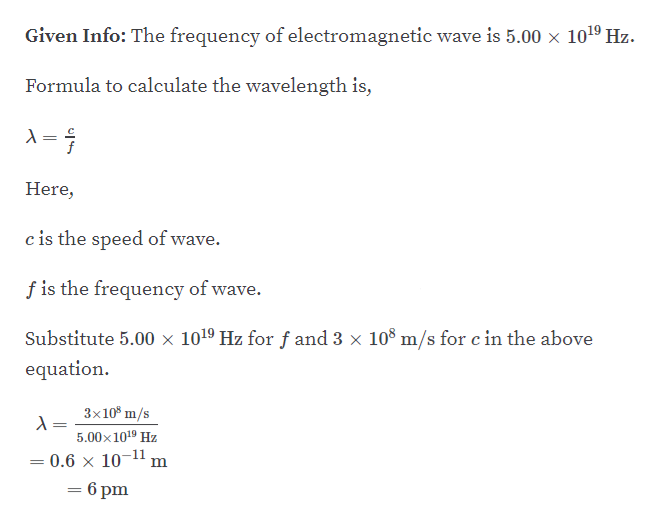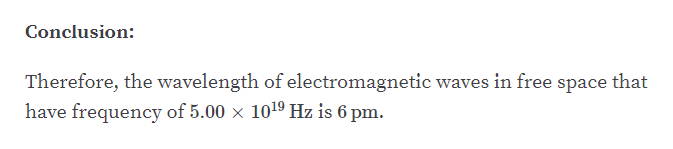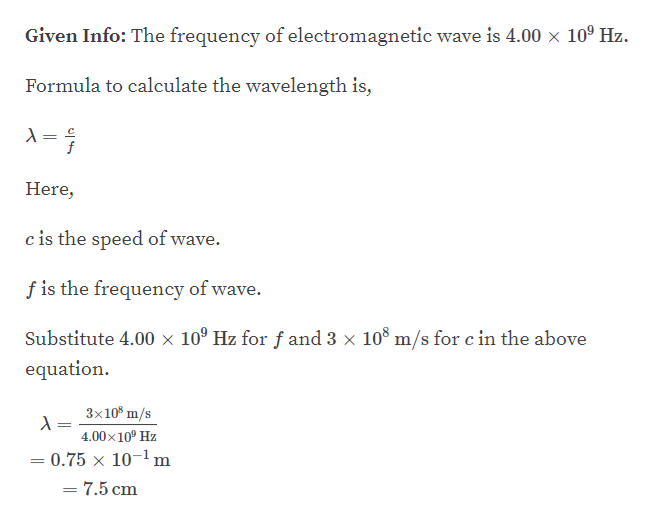# What are the wavelengths of electromagnetic waves infree space that have frequencies of (a) 5.00 x 1019 Hz and(b) 4.00 x 109 Hz?

Question
1 views

What are the wavelengths of electromagnetic waves in
free space that have frequencies of (a) 5.00 x 1019 Hz and
(b) 4.00 x 109 Hz?

check_circle

Step 1

Part A:help_outlineImage TranscriptioncloseGiven Info: The frequency of electromagnetic wave is 5.00 x 10o1º Hz. Formula to calculate the wavelength is, Here, c is the speed of wave. f is the frequency of wave. Substitute 5.00 × 1019 Hz for f and 3 x 10° m/s for c in the above equation. 3x10° m/s 5.00x1019 Hz 0.6 × 10-11 = 6 pm fullscreen
Step 2help_outlineImage TranscriptioncloseConclusion: Therefore, the wavelength of electromagnetic waves in free space that have frequency of 5.00 x 1019 Hz is 6 pm. fullscreen
Step 3

Part B:...help_outlineImage TranscriptioncloseGiven Info: The frequency of electromagnetic wave is 4.00 x 10º Hz. Formula to calculate the wavelength is, %3D Here, c is the speed of wave. f is the frequency of wave. Substitute 4.00 × 10º Hz for ƒ and 3 x 10° m/s for c in the above equation. 3x10° m/s %3| 4.00x10° Hz = 0.75 x 10-1 m = 7.5 cm fullscreen

### Want to see the full answer?

See Solution

#### Want to see this answer and more?

Solutions are written by subject experts who are available 24/7. Questions are typically answered within 1 hour.*

See Solution
*Response times may vary by subject and question.
Tagged in

### Other